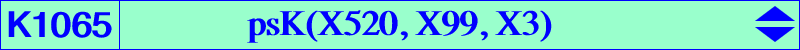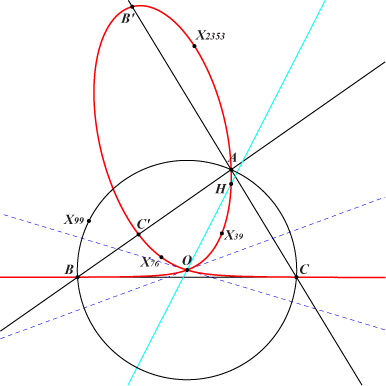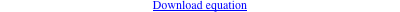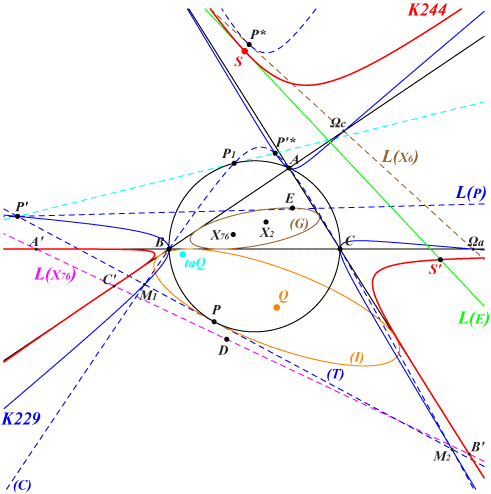too complicated to be written here. Click on the link to download a text file.X(3), X(4), X(39), X(76), X(2353), X(3926), X(5489), X(5562), X(14264), X(14357), X(14366), X(16391), X(23109), X(23110) vertices of the cevian triangle A'B'C' of X(99) Geometric properties :(based on notes by Peter Moses, 2018-08-28) Let P = p : q : r be a point with circumcevian triangle PaPbPc. Since the circumcircle (O) circumscribes both triangles ABC and PaPbPc, there must be a conic C(P) inscribed in these same triangles whose center is denoted Q. The coordinates of Q are : p (b^2 c^2 p (q + r) + a^2 (c^2 q^2 + b^2 r^2)) : : , and those of its anticomplement aQ are quite simple, namely : a^2 q r (c^2 q + b^2 r) : : or, equivalently, 1/p ( q/b^2 + r/c^2) : : . aQ can be seen as the barycentric quotient of the isogonal conjugate of P and the cevapoint of X(6) and P. Note that C(P) is a parabola if and only if P lies on K024 (further comments in Spanish here). Let 𝚹 be the mapping P → aQ. Important remark : 𝚹 maps any point P on the Lemoine axis onto X(76). See below for other properties of 𝚹. Now, any point P on another line (L) corresponds to a point aQ that lies on a nodal circum-cubic (K) passing through X(76). More details below. When (L) is the Euler line, the cubic (K) is K1065, the nodal psK(X520, X99, X3) with node the circumcenter O of ABC. Remarks : • When (L) is the line at infinity, the line X(1)X(3), the line X(23)X(385), the cubic (K) is K028, K1066, K1068 respectively. • Any P on (O) corresponds to a circumcevian triangle PaPbPc reduced to P and an inscribed conic tangent at P to (O). In this case, the point aQ lies on K244. Furthermore, there is a point P' on K229 = cK(#X6, X6) that corresponds to the same point aQ of K244. See below for more details and a figure. *** Properties of the cubic (K) Recall that (L) is a line with trilinear pole M = u : v : w ≠ X(6). 𝚹 maps (L) to the corresponding cubic (K), the nodal psK with : • pseudo-pivot P = t(M / X6) = isotomic conjugate of the M-Ceva conjugate of X6, • pseudo-isopivot Q = g(M^2) = isogonal conjugate of the barycentric square of M, • pseudo-pole Ω = P x Q = barycentric product of P and Q, • node N = Ω x M = barycentric product of Ω and M. *** An associated quartic (Q) Any line (L) as above is transformed under 𝚹 into the cubic (K) but there is a quartic (Q) that is also transformed into (K). For instance, when (L) is the line at infinity, (K) = K028, (Q) = Q098 and when (L) is the trilinear polar of X(83), (K) = K1068, (Q) = Q144. This quartic (Q) contains : • A, B, C with tangents concurring at T1 = M / X6 = tP = a^2 (-a^2 v w + b^2 u w + c^2 u v) : : , which is a node on (Q). • X(6). • the vertices Ka, Kb, Kc of the tangential triangle or anticevian triangle of X(6), with tangents concurring at T2 = (M^2 / X32) ÷ X6. • the vertices A', B', C' of the cevian triangle of M with tangents concurring at T3 = M x cgM = u^2 (c^2 v + b^2 w) : : . • the centers Ωa, Ωb, Ωc of the Apollonius circles, on the Lemoine axis, and the fourth point on this axis is T4 = a^2 (-2 a^2 v w + b^2 u w + c^2 u v) : : . • the common points A1, B1, C1 of a symmedian and the line passing through T1 and the corresponding Apollonius center. • the two (real or not) common points of (O) and (L). The two remaining points P1, P2 on (L) lie on the circum-conic passing through X(6) and T1, a conic which is the isotomic transform of the line through X(76) and P. • three other points Q1, Q2, Q3 on (O) – apart A, B, C and the two points on (L) as above – which also lie on pK(X6 x M, M), pK(X32, T1), pK(X6 x T1, X6) and more generally any pK with pole on pK(X1501 x M, X6 x M) and corresponding pivot on pK(X6 x M, M). In particular, for any M on the Kiepert hyperbola, one can always find such an isogonal pK and/or a pK with pivot X(2), with the special case mentioned above M = X(2) where we obtain the Thomson cubic K002 and the quartic Q098 passing through the vertices of the Thomson triangle. Remark : when M = X(110), (Q) splits into the Lemoine axis and K1067 = pK(X32, X110). More generally, for M on (O) hence for (L) passing through X(6), (Q) splits into the Lemoine axis and pK(X32, M). A remarkable property of (Q) : A variable line passing through the node T1 of (Q) meets (Q) again at two points M1, M2. The circle with diameter M1M2 is always orthogonal to the circumcircle (O) of ABC. See a figure in page Q145 for instance. *** Further properties of 𝚹, other associated quartics and related topics The 3rd degree mapping 𝚹 seen above has 6 singular points (A, B, C, the centers Ωa, Ωb, Ωc of the Apollonius circles) and 7 fixed points, one of them, say Z, being always real. These latter points are the common points – apart X(1670), X(1671) – of K100 and K141. This point Z has SEARCH = 2.192627440686386. Now, let F be a fixed point. The locus of P such that F, P and 𝚹(P) are collinear is a (circum) quartic Q(F) passing the 13 points above and also F, the vertices of the cevian triangle of F, the intersection of the line FX(76) and the Lemoine axis. Note that, when F = X(76), Q(F) splits into the Lemoine axis and K141 = pK(X2, X76). Other examples : Q(X2) = Q026, Q(X3) = Q147, Q(X4) = Q146. --- The 7 fixed points and the circular points at infinity are the base points of a pencil of circular cubics whose singular focus lies on the reflection about X(5) of the circumcircle (O). The real asymptote enveloppes the deltoid aH3. Obviously, one extra point S defines one and only one circular cubic of the pencil. Taking S = X(511) we obtain a cubic passing through X(1670), X(1671) hence the unique circular cubic of the pencil generated by K100 and K141. When S is one of the infinite points of K005, the circular cubic is a K+ hence it has its singular focus on its real asymptote.The special case P on (O) and the transformation 𝛕X* denotes the X(32)-isoconjugate of X i.e. the barycentric quotient X(32) ÷ X. L(X) is the trilinear polar of X. (G) is the inconic with perspector X(76), center X(141). Let P be a point on (O), (T) the tangent at P to (O), (I) the inconic of ABC tangent at P to (T) and (O), (C) the circum-conic which is the X(32)-isoconjugate of (T). (C) is tangent at P* to the Lemoine axis L(X6). The isotomic conjugate D of P lies on the de Longchamps axis L(X76). As seen above, the center of (I) is Q, its perspector is taQ, and let S = aQ = 𝚹(P) which lies on K244. (T) and (C) meet at M1, M2 on K229. The tangent (T) meets K229 again at P' also on the line L(P) and harmonic conjugate of P in M1, M2. For any P on (O), 𝚹(P') = 𝚹(P) = S. The line passing through P' and P'* is tangent at P1 to (O). S' = 𝚹(P1) is the tangential of S on K244. Obviously, the third point of K229 on this line is also mapped onto S' under 𝚹. The line SS' is the trilinear polar of a point E on (G).Note that the transformation 𝛕 that sends any point P in the plane to the intersection P' of the trilinear polar of P and the polar of P in (O) is given by : 𝛕 : P = u : v : w → P' = u^2 (c^2 v - b^2 w) : v^2 (a^2 w - c^2 u) : w^2 (b^2 u - a^2 v). 𝛕 maps the circum-conic with perspector Z onto the cubic cK(#Z, X6), an unicursal cubic with node Z and passing through the centers of the Apollonius circles. As already said, 𝛕 maps (O) onto K229. When Z = X(1), the cubic is K635. The nodal tangents at Z are perpendicular if and only if Z lies on K192 = nK(X6, X6, X110) which contains X(110), X(523) corresponding to the cubics K147 = cK(#X110, X6), K1072 = cK(#X523, X6) respectively. In this latter case, the nodal tangents are the line at infinity and the orthic axis.Generalization of the transformation 𝛕Let F = p : q : r and P = u : v : w be two distinct points not lying on the sidelines of ABC. Let (K) be the unicursal cubic cK(#F, P) with node F and root P. We define the generalized transformation 𝛕P as follows. If X is not P and if T is the trilinear pole of the line PX then X' = 𝛕P (X) is equivalently : • the intersection of the trilinear polar of X and the polar of X in the circum-conic C(P) with perspector P, • the T-Ceva conjugate of X, • the crosspoint of T and X (i.e. the pole of the line TX in the circum-conic passing through T and X). This transformation is given by 𝛕P : X = x : y : z → X' = x^2 (w y - v z) : y^2 (u z - w x) : z^2 (v x - u y). 𝛕P transforms the circum-conic C(F) with perspector F into the cubic (K) = cK(#F, P) and conversely, any cK can be characterized in this way. Obviously, when P = X(6), C(P) is the circumcircle which is transformed into cK(#F, X6) as seen above with 𝛕.